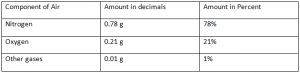Percentage: Means Of Comparing Quantities

In our daily life, we handle lots of situations, quantities, etc. Sometimes we have to measure and compare these quantities. The comparison is a common activity among us. Sometimes we compare our height, sometimes marks, sometimes speed, sometimes distance, and so many. This list of quantities extends. We can only compare two same quantities i.e. the height of a person cannot be compared with the age of another person. Hence, there should be always a common reference point for comparison. Similarly, we need a standard measure or way for comparing quantities. The percentage is one among them. Let’s learn how to compare two quantities in terms of percentage.

PERCENTAGE & COMPARING QUANTITIES

The term percentage or percent is not new to us. It is a very common word; we generally used to calculate our rank in the class. We always compare our mark and performance in percentage. It is easy to remember percentage than the total mark obtained in exams. Thus, we can assume percent is a much easier way for comparing quantities. But, what does the term percentage exactly means?

The term percent originated from ‘per centum’, a Latin word. It means per hundred. By definition, percent is a fraction with the denominator 100. It is denoted by the symbol % which means hundredths too.

For example, if you have 80% marks, it means mark you have obtained is equivalent to 80 out of hundred. This can be represented in decimals as follows:

Comparatively, percent is much better and easier method than any other method of decimals, fractions, and ratios. We know the air in our atmosphere is a mixture of gases. On comparison of the amount of different gases present in 1 gram of air, we can observe percentage is the easiest way for comparison.Thus, we can use this system of a percent to compare two quantities. But as mentioned above, comparing quantities must always be in the same unit.

This was just a brief discussion on percentage. Now get the detailed solutions to the questions of the NCERT Books for the chapter Comparing Quantities only at BYJU’S.